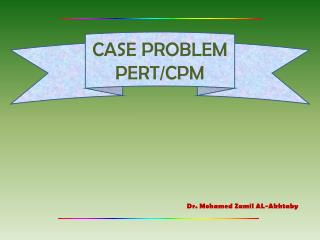DownloadDownload PresentationCASE PROBLEM PERT/CPM

# CASE PROBLEM PERT/CPM

Download Presentation## CASE PROBLEM PERT/CPM

- - - - - - - - - - - - - - - - - - - - - - - - - - - E N D - - - - - - - - - - - - - - - - - - - - - - - - - - -
##### Presentation Transcript

1. CASE PROBLEMPERT/CPM Dr. Mohamed Zamil AL-Akhtaby

2. CASE PROBLEM List of the activities

3. The optimistic, most probable and pessimistic time (in week)

4. Question (a)1 • R.C Coleman’s top management established a required 40-week completion time for the project. Can this completion time be achieved?

5. D I A START K FINISH C E F H J B G Project Network

6. Expected Time • Optimistic time (a) • The minimum activity time if everything progresses ideally • Most probable time (m) • The most probable activity time under normal conditions • Pessimistic time (b) • The maximum activity time if significant delays are encountered • Expected time (t) • The average time for the activity

7. Expected Time In Weeks

8. D 13 25 12 17 29 A 0 6 6 3 9 I 29 36 7 32 39 START K 39 43 4 39 43 C 9 13 4 9 13 E 13 23 10 13 23 F 23 29 6 23 29 H 29 35 6 29 35 FINISH B 0 9 9 0 9 J 35 39 4 35 39 G 13 21 8 21 29 Project Network (Earliest Start, Finish Times, Latest Start and Finish Times)

9. Activity Slack Time

10. Critical Path: B-C-E-F-H-J-K Project Completion Time: 43 weeks • R.C Coleman’s required 40-week completion time. This completion time can’t be achieved

11. Question (a)2 Include probability information in your discussion. What recommendations do you have if the 40-week completion time is required?

12. Variance (2) • used to describe the dispersion or variation in the activity time values

13. Variance of Each Activity

14. Variance in the project completion time (2) 2 = 2B + 2C + 2E + 2F + 2H + 2J + 2K = 2.78 + 0.44 + 1.00 + 0.44 + 0.44 + 0.11 + 0.44 = 5.65 Standard deviation ()  = √5.65 = 2.38

15.  = 2.38 Expected Completion Time 43 Time (weeks) Normal Distribution of Project Completion Time

16. Probability the project will meet the 40-week completion time  = 2.38 40 43 Time (weeks)

17. At T ≤ 40 z = (40 – 43) / 2.38 = 1.26 using table, α = Pr (T ≤ 40) = 0.1038 Pr (T ≤ 40) = 10.38% • We find that the probability of the project meeting the 40-weeks deadline is 10.38%. Thus, R.C Collman have a low chance if they want the project completed at 40-weeks

18. Question (b) • Suppose that management request that activity times be shortened to provide an 80 percent chance of meeting the 40-week completion time. If the variance in the project completion time is the same as you found in (a), how much should the expected project completion time be shortened to achieved the goal of an 80 percent chance of completion within 40 weeks?

19. Expected completion time to achieve 80% chance of completion within 40 weeks  = 2.38 X Time (weeks) 40

20. Pr (T ≤ 40) = 0.8000 Pr (T ≤ 40) = 1 - α α = 0.2 using table, z (T ≤ 40) = 0.84 (40 – x) / 2.38 = 0.84 x = 38 • It shows the project has to be shortened to 38 weeks to achieve the goal of an 80 %chance of completion within 40 weeks.

21. Question (c) • Using the expected completion times as the normal times and the following crashing information, determine the activity crashing decisions and revised activity schedule for the warehouse expansion project

22. Crash Time and Cost’s Information

23. Maximum possible reduction in time, Mi (3) with i = expected time for activity I ’i = time for activity i under maximum crashing Crash cost per unit of time, Ki with Ci = cost for activity I C’i = cost for activity i under maximum crashing

24. Activity Crashing Decision

25. Thank you